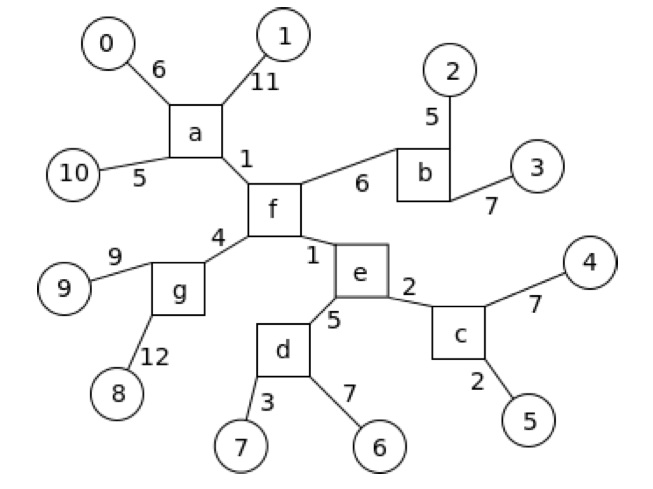# #234. 【IOI2015】Towns### 任务

• hubDistance(N, sub)
• N: ⼩城镇的数⽬。
• sub: ⼦任务编号（详⻅⼦任务描述部分）。
• sub是 $1$ 或者 $2$ 时，该函数返回 $R$ 或 $-R$ 均可。
• sub⼤于 $2$ 时，如果存在平衡的中⼼城市，该函数返回 $R$，否则返回 $-R$。

### ⼦任务

113$\frac{n(n - 1)}{2}$
212$\lceil \frac{7n}{2}\rceil$
313$\frac{n(n - 1)}{2}$
410$\lceil \frac{7n}{2}\rceil$每个大城市都刚好与 $3$ 个定居点连接
513$5n$
639$\lceil \frac{7n}{2}\rceil$

### 实现细节

int hubDistance(int N, int sub);

### 评测方式

• 第 $1$ ⾏：⼦任务编号和测试点的数⽬。
• 第 $2$ ⾏：$N_1$，第⼀个测试点中⼩城镇的数⽬。
• 接下来的 $N_1$ ⾏：第 $i$ ⾏（$1 \le i \le N_1$）第 $j$ 个（$1 \le j \le N_1$）数字是⼩城镇 $i - 1$ 到⼩城镇 $j - 1$ 的距离。
• 随后是下⼀个测试点的数据，格式与第 $1$ 个测试点的数据相同。

1 1
11
0 17 18 20 17 12 20 16 23 20 11
17 0 23 25 22 17 25 21 28 25 16
18 23 0 12 21 16 24 20 27 24 17
20 25 12 0 23 18 26 22 29 26 19
17 22 21 23 0 9 21 17 26 23 16
12 17 16 18 9 0 16 12 21 18 11
20 25 24 26 21 16 0 10 29 26 19
16 21 20 22 17 12 10 0 25 22 15
23 28 27 29 26 21 29 25 0 21 22
20 25 24 26 23 18 26 22 21 0 19
11 16 17 19 16 11 19 15 22 19 0